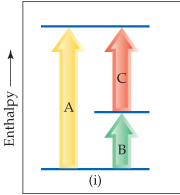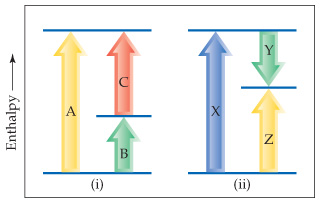# Problem: Consider the two diagrams below.Based on (i), write an equation showing how HA is related to HB and HC.

###### FREE Expert Solution

The chart shows the enthalpy (H) change A, B and C.94% (369 ratings)###### Problem Details

Consider the two diagrams below.Based on (i), write an equation showing how HA is related to HB and HC.